Torsional vibrations - what they are, and how to analyze them

As you may remember, the previous post covered the most significant types of vibrations in electrical machines. First on the list were torsional vibrations, for a reason.

Now, this post goes deeper into the details. We’ll recap the meaning of torsional vibrations, and see little of where and why they might occur. Next, we’ll take a brief look on the mathematics, in case you ever need to analyze something yourself. (Fear not – it’ll by much simpler than my usual stuff). And finally, some practical implications are discussed.

### Very basics

Let’s begin with a prototypical example: the two-mass system pictured below.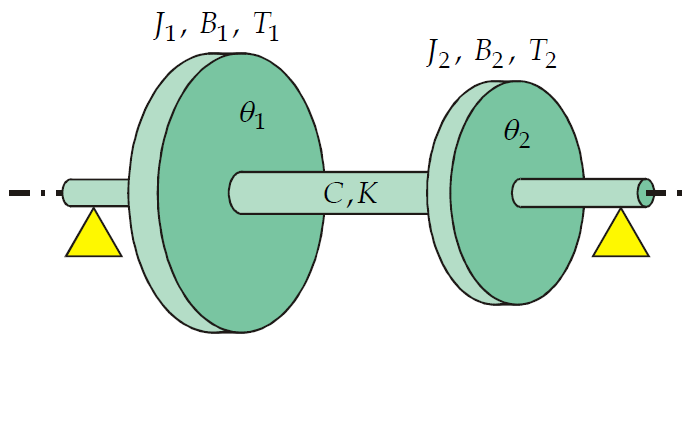We have two masses of inertia, connected by a shaft. For example, the smaller mass might be the rotor of an induction machine, driving a pump (the larger mass). The shaft of the two-mass system would then correspond to the coupling between the motor and the pump. So not the shaft of the motor itself, necessarily.

Now, let’s denote the rotation angles of the masses by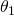and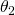, and their masses of inertia by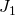and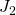. Now, neglecting all external torques and all damping, the system is governed by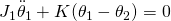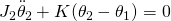As you probably know, the double dot notation is simply a shorthand for acceleration.

In other words, what we have are two terms related to torque. The first ones – the acceleration terms – relate to the torques needed for the speeding up or slowing down the rotating masses.

The second terms, on the other hand, denote the torque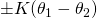provided by the twisting shaft. Indeed, the term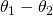is often called the torsion angle of the shaft.

And correspondingly, torsional vibrations refer to oscillations in the magnitude in this angle. And of course, oscillations in the anglesandof the masses themselves, by extension.

##### Natural frequency

Now, applying a little math yields us the natural frequency of the above system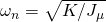where the equivalent mass of inertia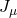is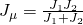.

##### Some implications

So, what’s the importance of the natural frequency? Well, as you should now, excitation (torque) at or near the natural frequency will generate large oscillations. Infinite, in fact, assuming the excitation perfectly matches the natural frequency and that there really isn’t any damping at all.

Even for realistic systems, having something excite the natural vibration is generally a very bad thing. It will generate torsional oscillations between the motor and the load. And as mentioned in the previous post, these oscillations usually don’t generate much noise or other symptoms. Thus, the first sign of them may often be something breaking down in the system.

Obviously, that situation should be avoided. But what could be exciting said vibrations? Well, either the motor or the pump, obviously.

The output torque of the motor is never perfectly smooth. Instead, there’s some ripple. So, what’s important in this case, is checking that the spectrum of the torque plays alright with the mechanical system.

Likewise, the load torque often varies as well. For example, pumps and impellers usually have torque ripple at the rotation frequency times the number of blades. The same applies to anything with a propeller, for that matter.

Furthermore, combustion engines typically exhibit large torque variations. So, owners of generator systems beware.

Finally, practically any kind of system will have some torque excitation at the rotation frequency and twice that, due to mechanical asymmetries (e.g. misalignment).

### Analysing more complex problems

As you can see, the sources of torque excitation are numerous. And, you should check that none of them is too close to the natural frequency of the system. That alone can be quite a bit of work.

But, things can get even more complex than this.

##### Damping

First of all, realistic systems obviously have some damping – otherwise they would oscillate forever. In a two-mass system, it can be shown that the natural frequency of a damped system is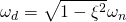,

where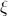is the damping ratio. For a system without any actual dampers, the ratio is usually small, on the order of a few percent. In that case, the damped and undamped natural frequencies are almost the same.

##### Larger systems

While a two-mass system – damped or not – can be analysed with pen and paper only, this is usually not the case for larger systems.

But what are larger systems in the first place? For example, this: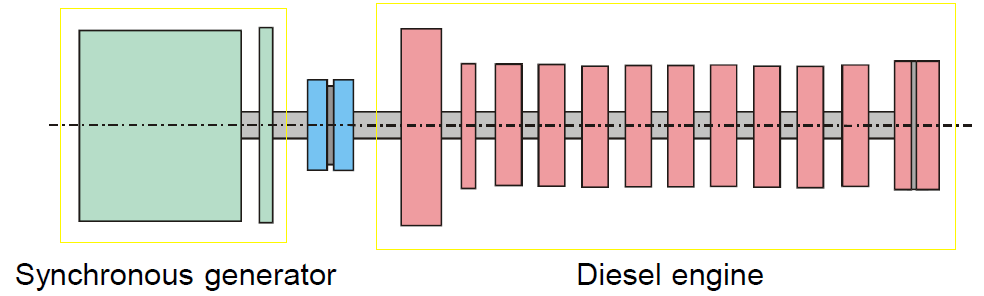Lumped model for a large torsional system. Screenshot from the Special Course on Electromechanics.

In other words, a generator driven by a diesel engine as pictured above. The generator itself could be reduced to a single rotating mass, but the engine usually cannot. This is because each piston, its connecting rod, and the piston-specific counterweight on the crankshaft, add up to a relatively significant moment of inertia when reduced on the shaft. Thus, a 16-cylinder diesel engine usually has to be analysed as a 17-mass system, the flywheel being the 17th one.

Add to that the generator, and we have 18 masses. Pen and paper suddenly seem quite insufficient.

Luckily, computers rush to the resque. Indeed, each masson the system is governed by an equation of type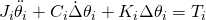.

In other words, we have a generalized version of the two-mass system earlier. This time, also the viscous damping term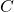is included.

(The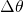symbols are a shorthand of mine. They simple represent the angle difference between adjacent masses, for instance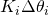being in reality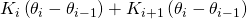. In other words, the torsion acting upon massby the adjacent massesand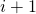, via the shaft segments in between. The same notation applies to the damping term, too.)

In our example, we would have 18 such equations. However, all of these could be compactly presented in matrix form like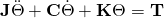by collecting all the terms of the individual equations into matrices.

##### Natural frequencies of the larger problem

From the matrix-formed problem, natural frequencies can easily be obtained with a computer. As you might know from basic mechanics, the key is to assume that all angles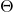oscillate in a sinusoidal fashion at the angular frequency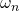with the amplitudes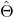. This yields the equation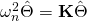when damping is ignored. This is a so-called generalized eigenvalue problem, meaning you getandwith pretty much any numerical software or library you like. I prefer Matlab.

If damping is taken into account, we get something a little more complex, but which can still be solved just as easily.

### Conclusion

Torsional vibrations can often be analysed by lumped models. This is usually the case when we have large separate rotating masses, connected by a relatively thin shaft. So, analysis the rotor of a long turbogenerator would probably have to be done by different means.

Regardless of the exact methodology, natural frequencies are probably the most interesting aspect. After all, having some torque excitation close to a natural frequency usually results in bad stuff(tm).

Finding the natural frequencies usually boils down to an eigenvalue problem. For small systems, this can be done by hand, but for larger ones computers are your best friend.

Next post: the interaction between torsional vibrations and machine torque.

-Antti

Need help with electric motor design or design software? Let's get in touch - satisfaction guaranteed!Torsional Vibrations in Electrical Machines